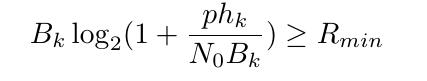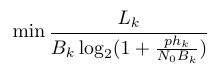# How to represent this expression in CVXIf there anyone know how to represent this expression in CVX？Only B_k is variable . Thanks advance！

`-rel_entr(Bk,Bk+p*hk/N0)/log(2) >= Rmin`

In general, `x*log(1+y/x)` can be formulated as `-rel_entr(x,x+y)` .presuming `x` is affine and `y` is concave (note that a numerical constant is concave).

Thanks for your help. I am very sorry that there is another problem :is that can achieve in CVX? Only B_k is variable too. And constraint of B_k is :0<=B_k <=B_max(a constant)You can apply `inv_pos`, because it accepts a concave argument.

``````minimize(Lk*inv_pos(-rel_entr(Bk,Bk+p*hk/N0)/log(2)))
0 <= Bk <= B_max
``````

help inv_pos

inv_pos Reciprocal of a positive quantity.
inv_pos(X) returns 1./X if X is positive, and +Inf otherwise.
X must be real.

`````` For matrices and N-D arrays, the function is applied to each element.

Disciplined convex programming information:
inv_pos is convex and nonincreasing; therefore, when used in CVX
specifications, its argument must be concave (or affine).
``````

You may benefit from a careful reading of the CVX Users’ Guide http://cvxr.com/cvx/doc/ , as well as the Mosek Modeling Cookbook https://docs.mosek.com/modeling-cookbook/index.html

Hello @Mark_L_Stone , is this approach still applicable when you have A+N_0B_k in the log function instead of N_0B_k? A is constant. Thanks in advance.

Presuming A>= 0, the same basic idea can be used, with some extra complication: The expression after the lattermost `= ` can be entered in CVX

``````x*log(1+y/(x+A)) = (x+A)*log(1+y/(x+A)) - A*log(1+y/(x+A))
= -rel_entr(x+A,x+A+y) - A*(rel_entr((x+A)/y,(x+A)/y+1) + rel_entr((x+A)/y+1,(x+A)/y))
``````

If x and y are both variables, the decomposition in the first line does not result in a formulation which can be entered in CVX, although it is still mathematically correct. That is because `A*log(1+y/(x+A`) is neither convex nor concave jointly in x; and y, and therefore can;'t be reformulated for CVX, even though `x*log(1+y/(x+A)`) is jointly concave in x and y.

And of course you can apply `inv_pos` to this, if you want the reciprocal of this expression. And that would be convex.

1 Like